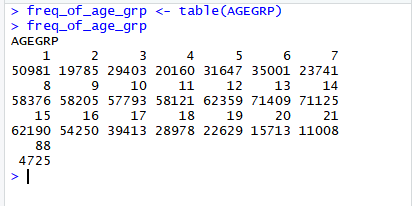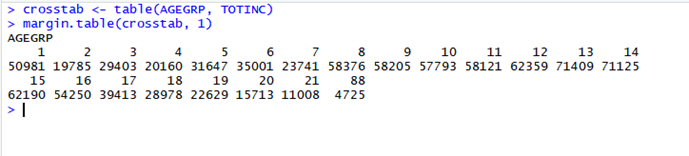# Learn R

This guide focuses on transformation and cleaning functions in R that are especially useful for working with tabular datasets.

## Frequency distribution of a single column

The frequency distribution of a data frame can be found using the table() function. Below is the frequency distribution of column AGEGRP.

`myvars <- c(“AGEGRP”, “TOTINC”, “WEIGHT”)`
`new_data_frame <- df[myvars]`## Crosstabulations (2- way frequencies)

To generate 2 way frequency table (or cross tabulation) pass 2 columns to the table() function. For the example below, a crosstab dataframe is created using table() and then margin.table is used to get the frequencies of Total Income by Agegroup.

`myvars <- c(“AGEGRP”, “TOTINC”, “WEIGHT”)`
`new_data_frame <- df[myvars]`The general form of the main functions for cross tabulations are:

`mytable <- table(A,B) # A will be rows, B will be columns`
`margin.table(mytable, 1) # B frequencies (summed over A)`
`margin.table(mytable, 2) # A frequencies (summed over B)`
`prop.table(mytable) # cell percentages`
`prop.table(mytable, 1) # row percentages`
`prop.table(mytable, 2) # column percentages`

## Liaison LibrarianMartin Morris
Contact:
Schulich Library of Physical Sciences, Life Sciences and Engineering
Macdonald-Stewart Library Building
809 rue Sherbrooke Ouest
Montréal, Québec H3A 0C1
(514) 398 8140
Website Skype Contact: martinatmcgill